# 013 Postgres command line : psql : Using ST_Within function to build junction tables to compare 2 separate polygon tables

First off let us create a new database to hold our examples in.

```CREATE DATABASE stwithindb;
```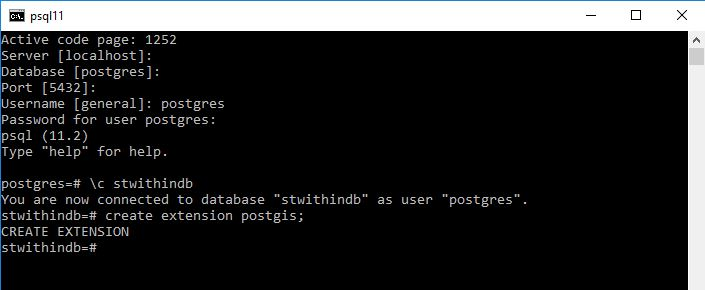Lets create two tables one called fields and one called plots

```CREATE TABLE
t00001fields
(
pkid serial primary key,
fieldname varchar(50),
geom geometry(polygon,27700)
)
;
CREATE TABLE
t00002Plots
(
pkid serial primary key,
plotname varchar(50),
geom geometry(polygon,27700)
)
;```

Now lets go to QGIS connect to the PostGIS instance add the tables and create some test data manually.

Here I have added fields in green with bold number labels and plots in brown with smaller number labelling. The numbers represent the pkid fields.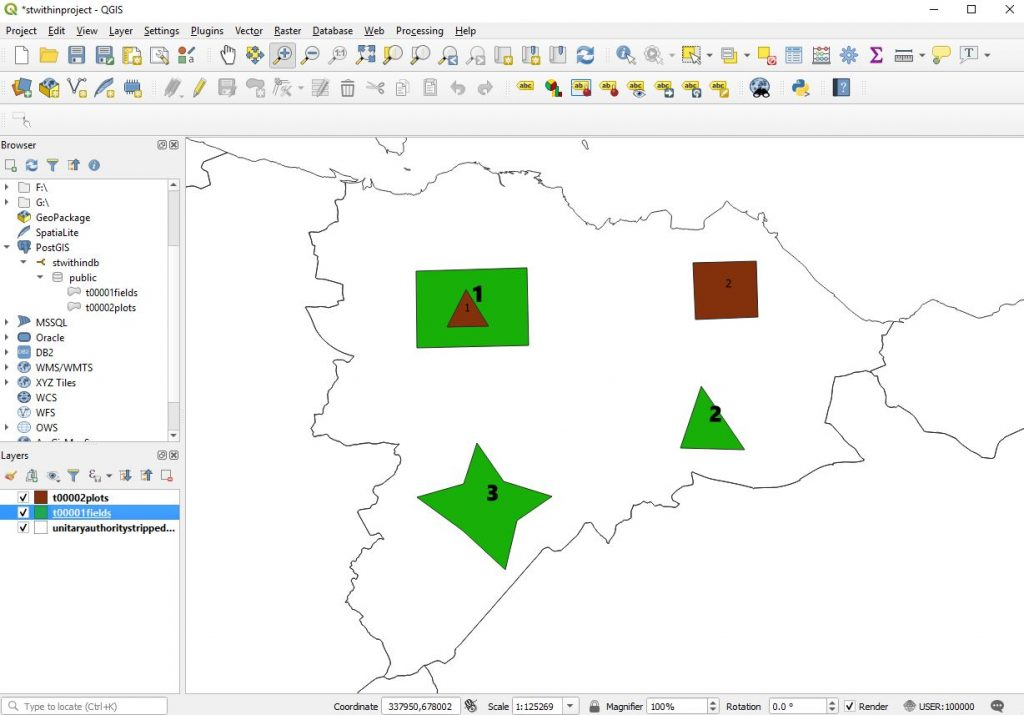Now here I can quickly run a query to identify the plots that are in fields

```SELECT t00002plots.pkid
FROM
t00002plots,
t00001fields
WHERE
ST_WITHIN(PUBLIC.T00002PLOTS.GEOM, PUBLIC.T00001FIELDS.GEOM);```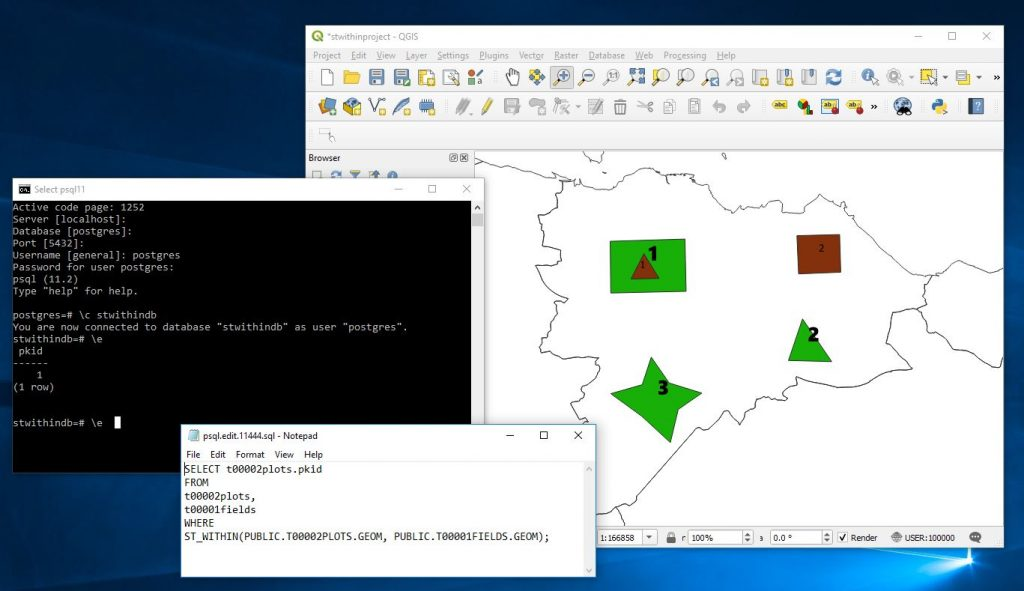And it correctly identifies that plot 1 is within the fields layer.

But what would be great in an application is to have some kind of junction table that individual master records could display their children on. For this we need a junction table that links between the field and plots table showing the pkids from each.

```SELECT t00002plots.pkid as Plotspkid,t00001fields.pkid as Fieldspkid
FROM
t00002plots,
t00001fields
WHERE
ST_WITHIN(PUBLIC.T00002PLOTS.GEOM, PUBLIC.T00001FIELDS.GEOM);```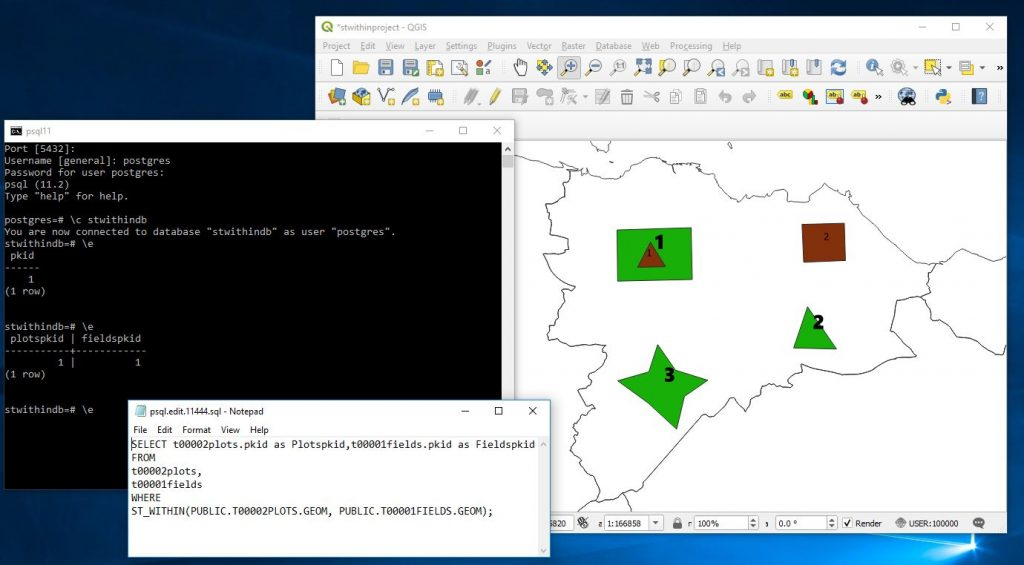Now I will move plot 2 into field 3 and rerun the above.

The layer now looks likeand running the former query we get.Now its possible to either create a junction table to hold this information..

eg

```CREATE TABLE t00010fieldplotjunction AS
SELECT t00002plots.pkid as Plotspkid,t00001fields.pkid as Fieldspkid
FROM
t00002plots,
t00001fields
WHERE
ST_WITHIN(PUBLIC.T00002PLOTS.GEOM, PUBLIC.T00001FIELDS.GEOM);```

or we can create a view that will constantly calculate this everytime it is seen

```CREATE VIEW v001FieldPlotJunction AS
SELECT t00002plots.pkid as Plotspkid,t00001fields.pkid as Fieldspkid
FROM
t00002plots,
t00001fields
WHERE
ST_WITHIN(PUBLIC.T00002PLOTS.GEOM, PUBLIC.T00001FIELDS.GEOM);```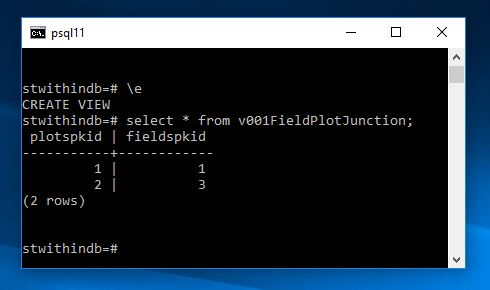Now if I add a few more plots and fields and then pull up the view we shall see that everything has been adjusted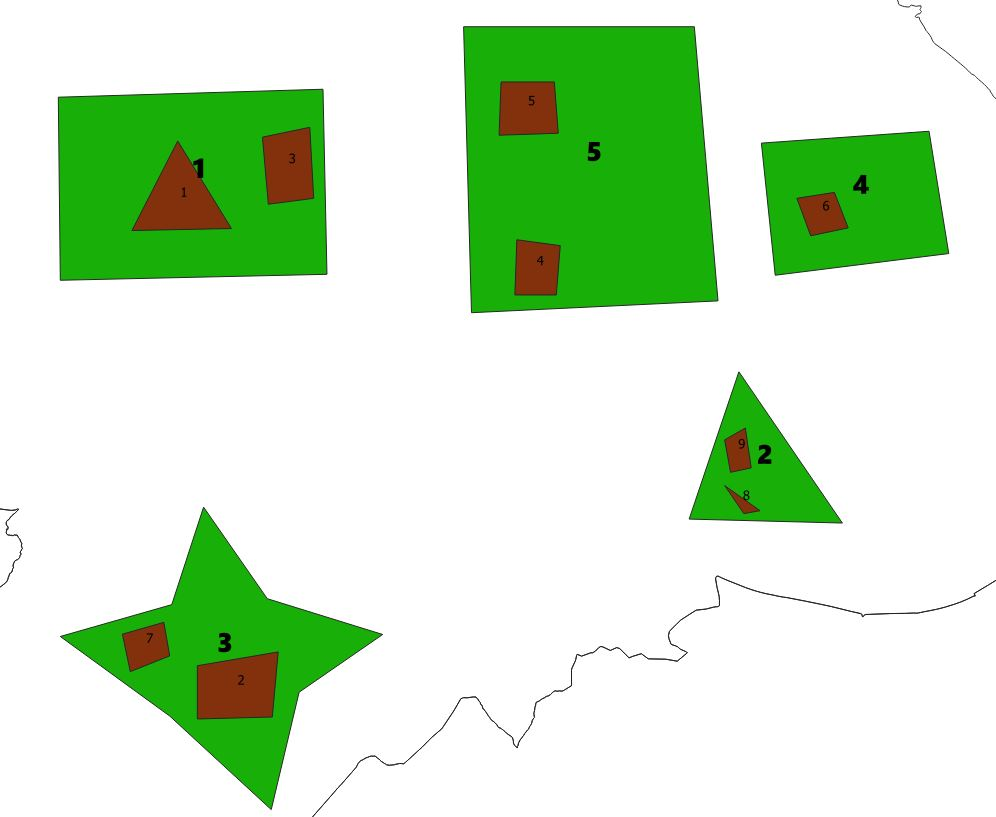and running the view we now get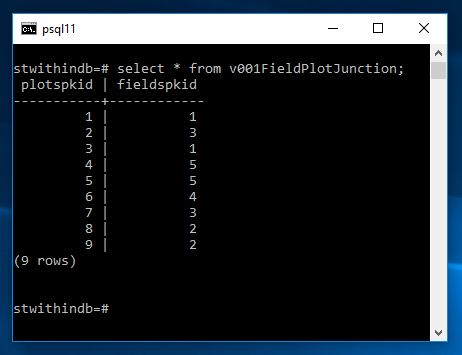In some circumstances this calculation may be expensive so we may wish to run and create a junction table overnight other times we may be happy to do it fully dynamically. Of course in a front end you could query and filter such that only one record was compared against the fields plot at anytime. Very useful nonetheless.